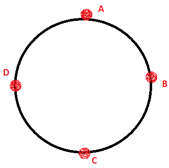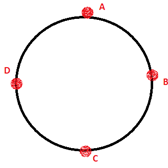Physics
Easy

Question

# A girl is sitting in a merry-go-round.  The time period of circular motion of the merry-go-round is 8 sec and it forms a circle of 40 m radius. What is the speed of rotation?

## 30.9 m/s810.4 m/s480.34 m/s31.4 m/sHint:

## The correct answer is: 31.4 m/s

Physics

Physics

Physics

### What is the direction of the velocity at point mentioned if the ball is revolving anticlockwise?At point BPhysics

Physics

Physics

### The time taken for one complete circle is called the _____.Physics

Physics

Physics

### A boy moves a tied stone above his head in circles, what is the time period of circular motion if it forms a circle of 20 cm radius. The speed of rotation is 23 m/s.Physics

Physics

Physics

### What is the direction of the velocity at point mentioned if the ball is revolving clockwise.At point CPhysics

Physics

Physics

### What is the velocity formula for uniform circular motion?#### With Turito Foundation.#### Get an Expert Advice From Turito.